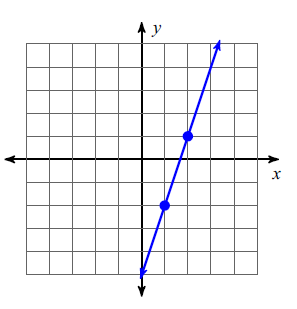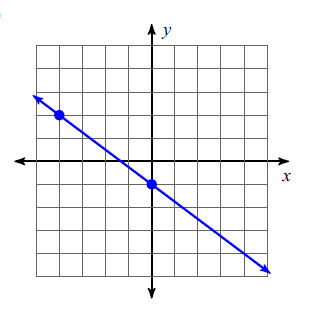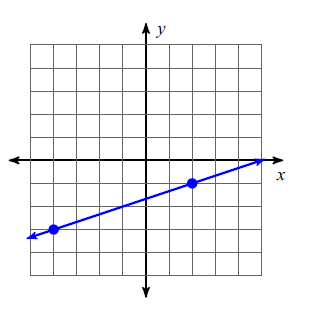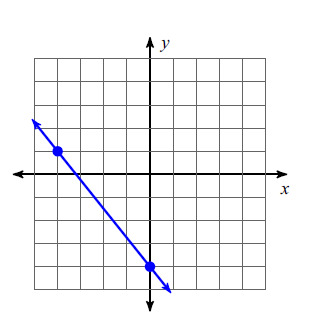Writing In Point-Slope Form
Identify the Point & Slope from Equations
Point-Slope Form
from Graphs
Convert to Slope-Intercept Form
Slope/Y-intercept
100

Write the point-slope form of the equation of the line through the given point with the given slope.

Point: (2 , 3)

M: 1/4

y - 3 = 1/4(x - 2)

100

Identify the Point and Slope.

y - 3 = 2(x -11)

(11, 3)

m = 2

100

Write an equation in Point-Slope Formy - 1 = 3(x - 2)

OR

y + 2 = 3(x - 1)

100

The slope-intercept form of this equation

y - 5 = 2(x + 4)

What is

y = 2x + 13

100

In the equation y=mx+b    "b" represents

What is

the y-intercept

200

Write the point-slope form of the equation of the line through the given point with the given slope.

Point: (-4 , -4)

Slope: 5

y + 4 = 5(x + 4)

200

Identify the Point and Slope.

y - 6 = 0(x + 7)

(-7, 6)

m = 0

200

Write an equation in Point Slope Form.y - 2 = -3/4(x + 4)

OR

y + 1 = -3/4(x - 0)

200

The slope-intercept form of this equation

y - 3 = 1/2(x + 10)

What is

y = 1/2x + 8

200

Slope is _______ over _______

What is

delta y over delta x,

change in y over change in x

rise over run,

y2-y1 all over x2-x1

300

Write the point-slope form of the equation of the line through the given points using the FIRST point.

(-3 , -3) and (-5, 4)

y + 3 = -7/2(x + 3)

300

Identify the Point and Slope.

y - 0 = -2/5(x + 14)

(-14 , 0)

m = -2/5

300

Write an Equation in Point Slope Form.y + 1 = 1/3(x - 2)

OR

y + 3 = 1/3(x + 4)

300

The slope-intercept form of this equation

y - 1 = -3/4(x - 0)

What is

y = -3/4x + 1

300

Y- intercept represents this

What is

The point at which the graph crosses the y axis.

400

Write the point-slope form of the equation of the line through the given points using the SECOND point.

(-5, 3) and (0, 3)

y - 3 = 0(x - 0)

400

Identify the Point and Slope.

y - 15 = -(x + 20)

(-20 , 15)

m = -1

400

Write an equation in Point Slope Form.y - 1  = -5/4(x + 4)

OR

y + 4  = -5/4(x - 0)

400

The slope-intercept form of this equation

y - 0 = 1/3(x + 9)

What is

y = 1/3x + 3

400

A vertical line has this slope

What is

undefined or no slope

500

Write the point-slope form of the equation of the line through the given points using the FIRST point.

(-2 , -3) and (2 , 3)

y + 3 = 3/2(x + 2)

500

Identify the Point and Slope.

y + 9 = 2/7(x - 0)

(0, -9)

m = 2/7

500

Write an equation in Point Slope Form.y + 2  = 2/5(x + 2)

OR

y - 4  = 2/5(x - 3)

500

The slope-intercept form of this equation

y - 5 = -(x + 10)

What is

y=-x-5

500

All Horizontal Lines have this slope

What is

0

Click to zoom